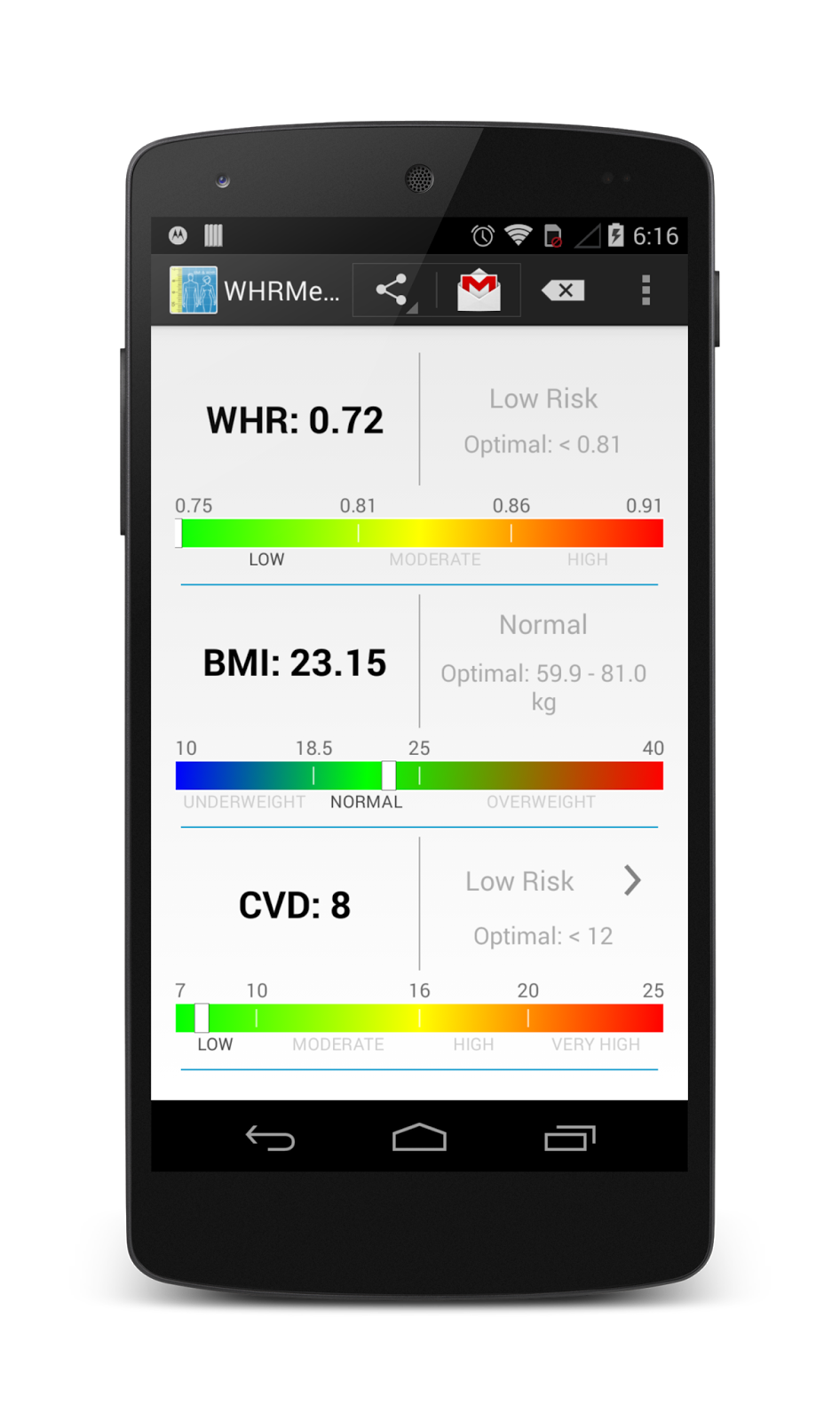## Saturday, July 5, 2014

### WHRMeter for Android: WHR, BMI and CVD calculations

WHRMeter is an Android app that calculates  Waist To Height Ratio (WHR) and health risk based on it, Body Mass Index (BMI) and estimates Cardiovascular Disease Risk (CVD).

Using Body Mass Index you can check your optimal weight for given height.Key Features:

• optimized for tablets
• imperial and metric units
• convert input values to different metric system
• instant WHR, BMI calculation and  CVD estimation
• show current weight condition (underweight, overweight, obesity level) and calculate optimal weight range
• presenting results in different forms
• show your results relative to a full scale of values for better understanding
• share your parameters (age, height, weight, etc.) and calculation results
• help section
• no permissions and pop-up ads